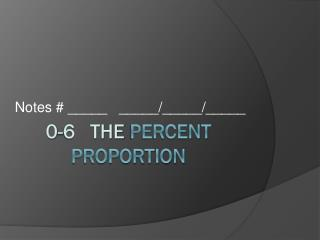DownloadDownload Presentation0-6 the Percent Proportion

# 0-6 the Percent Proportion

Download Presentation## 0-6 the Percent Proportion

- - - - - - - - - - - - - - - - - - - - - - - - - - - E N D - - - - - - - - - - - - - - - - - - - - - - - - - - -
##### Presentation Transcript

1. Notes # _____ _____/_____/_____ 0-6 the Percent Proportion

2. percent: a ratio that compares a number to 100 percent proportion : DECIMALS are ok on problems that involve %. If you have to round, round to the nearest tenth.

3. Use the percent proportion to find each number. What % of 56 is 18? 24% of 25 is what number? Ex 1 Ex 2

4. What % of 10 is 50? What % of 50 is 10? Ex 3 Ex 4

5. 38.25 is 45% of what number? 12 is 40% of what number? Ex 5 Ex 6

6. In a bag of party favors, 39 out of 60 are whistles. What percent of the part favors are whistles? Ex 7